Download Presentation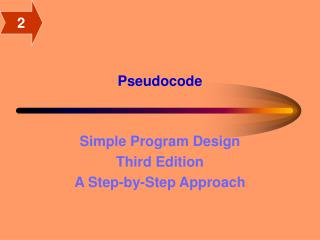Pseudocode

# Pseudocode - PowerPoint PPT Presentation

2. Pseudocode. Simple Program Design Third Edition A Step-by-Step Approach. 2. Objectives. To introduce common words and keywords used when writing pseudocode To define the three basic control structures as set out in the Structure TheoremI am the owner, or an agent authorized to act on behalf of the owner, of the copyrighted work described.
Download Presentation## Pseudocode

An Image/Link below is provided (as is) to download presentation

Download Policy: Content on the Website is provided to you AS IS for your information and personal use and may not be sold / licensed / shared on other websites without getting consent from its author.While downloading, if for some reason you are not able to download a presentation, the publisher may have deleted the file from their server.

- - - - - - - - - - - - - - - - - - - - - - - - - - E N D - - - - - - - - - - - - - - - - - - - - - - - - - -
Presentation Transcript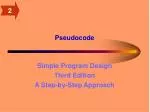2

### Pseudocode

Simple Program Design

Third Edition

A Step-by-Step Approach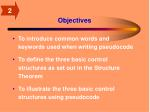2

Objectives
• To introduce common words and keywords used when writing pseudocode
• To define the three basic control structures as set out in the Structure Theorem
• To illustrate the three basic control structures using pseudocode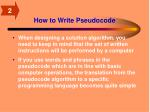2

How to Write Pseudocode
• When designing a solution algorithm, you need to keep in mind that the set of written instructions will be performed by a computer
• If you use words and phrases in the pseudocode which are in line with basic computer operations, the translation from the pseudocode algorithm to a specific programming language becomes quite simple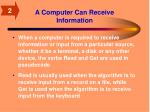2

A Computer Can Receive Information
• When a computer is required to receive information or input from a particular source, whether it be a terminal, a disk or any other device, the verbs Read and Get are used in pseudocode
• Read is usually used when the algorithm is to receive input from a record on a file, while Get is used when the algorithm is to receive input from the keyboard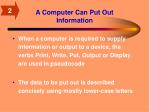2

A Computer Can Put Out Information
• When a computer is required to supply information or output to a device, the verbs Print, Write, Put, Output or Display are used in pseudocode
• The data to be put out is described concisely using mostly lower-case letters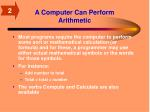2

A Computer Can Perform Arithmetic
• Most programs require the computer to perform some sort or mathematical calculation (or formula) and for these, a programmer may use either actual mathematical symbols or the words for those symbols.
• For instance:
• Add number to total
• Total = total + number
• The verbs Compute and Calculate are also available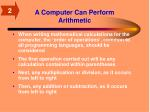2

A Computer Can Perform Arithmetic
• When writing mathematical calculations for the computer, the ‘order of operations’, common to all programming languages, should be considered
• The first operation carried out will be any calculation contained within parentheses
• Next, any multiplication or division, as it occurs from left to right
• Then, any addition or subtraction, as it occurs from left to right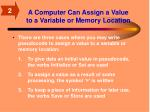2

• There are three cases where you may write pseudocode to assign a value to a variable or memory location:

1. To give data an initial value in pseudocode, the verbs Initialize or Set are used

2. To assign a value as a result of some processing, the symbol ‘=‘ is written

3. To keep a piece of information for later use, the verbs Save or Store are used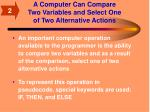2

A Computer Can Compare Two Variables and Select One of Two Alternative Actions
• An important computer operation available to the programmer is the ability to compare two variables and as a result of the comparison, select one of two alternative actions
• To represent this operation in pseudocode, special keywords are used: IF, THEN, and ELSE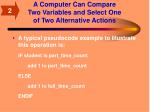2

A Computer Can Compare Two Variables and Select One of Two Alternative Actions
• A typical pseudocode example to illustrate this operation is:

IF student is part_time_count

add 1 to part_time_count

ELSE

add 1 to full_time_count

ENDIF2

A Computer Can Repeat a Group of Actions
• When there is a sequence of processing steps which need to be repeated, two special keywords (DOWHILE and ENDDO) are used in pseudocode
• The condition for the repetition of a group of actions is established in the DOWHILE clause, and the actions to be repeated are listed beneath it
• The format, indentation, and operation of DOWHILE and WHILE…DO are exactly the same2

The Structure Theorem
• The Structure Theorem forms the basic framework for structured programming
• It states that it is possible to write any computer program by using only three basic control structures that are easily represented in pseudocode:
• Sequence
• Selection
• Repetition2

Sequence
• The sequence control structure is the straightforward execution of one processing step after another
• We represent this construct as a sequence of pseudocode statements

statement a

statement b

statement c2

Sequence
• The sequence control structure can be used to represent the first four basic computer operations listed previously: to receive information, put out information, perform arithmetic, and assign values
• These instructions illustrate the sequence control structure as a straightforward list of steps written one after the other, in a top-to-bottom fashion2

Selection
• The selection control structure is the presentation of a condition and the choice between two actions
• The choice depending on whether the condition is true or false
• This construct represents the decision-making abilities of the computer and is used to illustrate the fifth basic computer operation, namely to compare two variables and select one of two alternate actions2

Selection
• In pseudocode, selection is represented by the keywords IF, THEN, ELSE and ENDIF:

IF condition p is true THEN

statement(s) in true case

ELSE

statement(s) in false case

ENDIF

• If condition p is true then the statement or statements in the true case will be executed, and the statements in the false case will be skipped2

Repetition
• The repetition control structure can be defined as the presentation of a set of instructions to be performed repeatedly, as long as a condition is true
• The basic idea of repetitive code is that a block of statements is executed again and again, until a terminating condition occurs2

Repetition
• It is written in pseudocode as:

DOWHILE condition p is true

statement block

ENDDO

• The initializing and subsequent incrementing of the variable tested in the condition is an essential feature of the DOWHILE construct2

Summary
• Six basic computer operations are listed in the text, along with pseudocode words and keywords to represent them.
• These operations are:
• To receive information
• Put out information
• Perform arithmetic
• Assign a value to a variable
• Decide between two alternate actions
• Repeat a group of actions
• The Structure Theorem